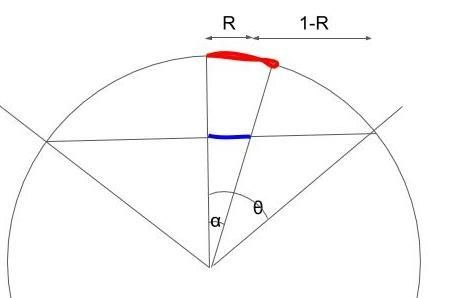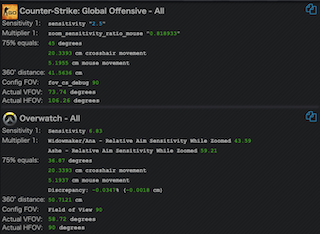# Math behind Monitor Distance Match

In Mouse Sensitivity | Same Aim - Different Game, there is a sensitivity conversion method called Monitor Distance Match. The method takes a number as parameter which represents the percentage of the match distance per the entire screen width so the general form is MMx which means "Monitor Distance Match in x%". This article explains the math behind the MMx calculation.

### Math

In MMx, the goal is to match the physical mouse movement to move the cursor in the same distance between two different FOVs so player transfers the same feeling of flicking the distance between games.In this figure, the R represents the ratio x. So the goal of MMx is to convert the sensitivity so the time to pass the red arc is the same between two games. Let do some math with this figure.

We can choose any number as the radius of the circle because the conversion ratio is our only concern. Let choose one here.

The length of the blue line (chord) isand the length of the arc is.

Now the sensitivity is the inverse of angle speed and the time to pass the arc iswhere L is the sensitivity.

Recall the goal is to have the same time to pass the arc then we get.

### Program

I wrote a small Python script to test the math.

import sys
import math
args = sys.argv
ratio = float(args)
fov0 = float(args) * 0.5 * math.pi / 180
fov1 = float(args) * 0.5 * math.pi / 180
sens0 = float(args)
alpha0 = math.atan(ratio * math.tan(fov0))
alpha1 = math.atan(ratio * math.tan(fov1))
sens1 = sens0 * alpha0 / alpha1
print(sens1)


Let's convert the 1000edpi in CSGO to OW in 90FOV using MM75 method.

\$ python3 mmx.py 0.75 106.26 90 41.5636
50.72858043985662

The result is very close to the number we get in mouse-sensitivity.com (50.71cm) so the math is proven to be correct.